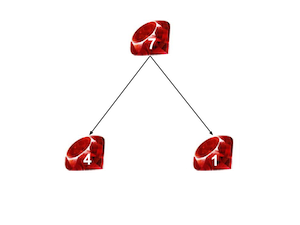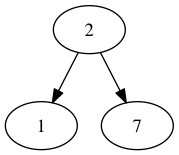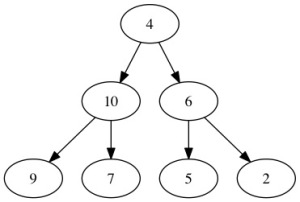# Heap Data Structure in Ruby

Ruby

Share:

#### Free JavaScript Book!Write powerful, clean and maintainable JavaScript.

RRP \$11.95The study of data structures and algorithms does not have a huge following in the Ruby community because we often ask “what’s the point?” In practice, we rarely have to implement classical structures such as stacks and queues. But, by learning about them, we can improve our ability to reason about code. More importantly, data structures are very interesting to study!

In this article, we’ll cover the “heap” data structure along with some of the associated algorithms. I’ll assume that you have a basic handle on asymptotic notation and Ruby.

## Motivation

We all have waaay too many emails. In an example where we’ve managed to assign every email in our inbox a priority or a key value (i.e. a number), we want to be able to do the following things:

1. Get the email with the largest key
2. Remove and get the email with the largest key

This will have to deal with a lot of emails, so we want these operations to be pretty fast, asymptotically speaking. What data structure should we use to achieve these goals? Turns out that by using a heap, we do Operation #1 in $$O(1)$$ time and Operation #2 in $$O(\log_2{n})$$.

## The Concepts

More specifically, we’ll be dealing with a “max heap”. From here on out, we’ll use the terms “max heap” and “heap” interchangeably. A max heap can be visualized as a partially complete binary like this:The thing that makes it special is that, for any node, the children of that node have keys smaller than or equal to that of the node. Note that in the figure above, the keys for each node are written within the node. Generally, heaps are actually stored as arrays. So our example above would look this:

[10, 4, 8, 2, 1, 7]

We create the array by going top to bottom and left to right through the binary tree representation and appending the node keys to an array. It is important to know that not all arrays are heaps. For example, take:

[2, 1, 7]

We can visualize that as:which is not a heap since $$7 > 2$$. Basically, a heap is an array with the special condition that, when visualized as a tree, the max heap property holds. In other words, the children of each node have key values smaller than that of the node itself.

## Constructing a Heap

The first problem that we have is our list of emails could have an arbitrarily ordered array of priorities that might not form a heap. How do we take this array and order it so that it forms a heap?

Well, that seems like a pretty complicated problem to tackle all at once, so let’s deal with a smaller version of it first. Let’s say we were given an array that was just one “swap” away from becoming a heap. So, we have some node v so that both its left and right subtrees are heaps but the subtree rooted at v is not a heap:We can resolve this issue recursively. First, switch the 4 and the 10. Then, we have nearly the same problem once again, just in a different place in the tree since $$9 > 4$$ and $$7 > 4$$, so we can solve it using the same method. We keep doing that until $$4$$ “sinks” to the bottom of the tree and becomes a leaf. Here’s how our approach will work:

fix_one_error(array, index):
1. If array[index] is a leaf node or is greater than both its children, return.
2. larger_index = the index of the larger child of the node at array[index]
3. Swap array[i] and array[larger_index]
4. fix_one_error(array, larger_index)

Alright, let’s implement the ideas in Ruby. First of all, we need a couple of methods to give us the indices of the children of a given node in the array representation:

class Heap
attr_accessor :heap_size, :array_rep
def left_child(index)
2*index + 1
end

def right_child(index)
2*index + 2
end

def left_child_key(index)
@array_rep[left_child(index)]
end

def right_child_key(index)
@array_rep[right_child(index)]
end
end

Second, we need some way to see if a given element is a leaf node or not:

class Heap
...
def leaf_node?(index)
return index >= @heap_size/2
end
...
end

Finally, we need to be able to tell if a node already satisfies the max heap property (in which case, we don’t need to keep fixing errors):

class Heap
...
def satisfied?(index)
@array_rep[index] >= left_child_key(index) and
@array_rep[index] >= right_child_key(index)
end
...
end

The details of how these four methods work aren’t incredibly important, but drawing out a few heaps with the array indices marked can make their inner mechanics pretty clear. Now, we can write out our algorithm in its full glory:

class Heap
...
def fix_one_error(index)
return if leaf_node?(index) || satisfied?(index)

left_child_key = @array_rep[left_child(index)]
right_child_key = @array_rep[right_child(index)]

larger_child = if left_child_key > right_child_key then left_child(index)
else right_child(index) end

@array_rep[index], @array_rep[larger_child] = @array_rep[larger_child],
@array_rep[index]

fix_one_error(larger_child)
end
...
end

Notice that we’ve followed the pseudocode/description very closely. Repeatedly swap until either the node satisfies the max heap property or it has floated down the tree to become a leaf node.

## Complexity of “Fix One Error”

At first glance, we might think that our fix_one_error algorithm is $$O(n)$$, but upon actually solving the recurrence we find that it is $$O(\log_2{n})$$. The intuitive reasoning is pretty clear: Each swap resulting from a call of fix_one_error takes $$O(1)$$ time and when we have $$n$$ elements, our tree has $$\log_2{n}$$ levels in it so we end up with $$O(\log_2{n})$$ as our time complexity.

## Finishing Up the Construction

But, we haven’t solved the whole problem of converting an arbitrary array into a max heap quite yet. So far, we’ve only solved the case where there is only one error. As it happens, we can repeatedly use the “one error case” to solve the whole problem!

First of all, notice that leaf nodes, by themselves, automatically satisfy the max heap property since they do not have any children. Now, consider the nodes that are one level above leaves, i.e. only have leaf nodes as children. These nodes can only be, at most, one swap away from satisfying the max heap property. This means we can use our fix_one_error function in order to make sure that all nodes that are one level above the leaves form heaps (or, satisfy the max heap property). Now, when we move up another level, we know that everything below satisfies the property, so we can use fix_one_error yet again! Essentially, starting from just above the leaf nodes, we can repeatedly apply fix_one_error in order to convert any array into a heap.

## Complexity of Heap Construction

We can answer pretty easily when asked for the time complexity of constructing a heap. With $$n$$ nodes, run fix_one_error on every node and the complexity for each is $$O(log_2{n})$$. Therefore, our heap construction algorithm should be $$O(n\log_2{n})$$, right?

While this is correct, it turns out that we can do better (i.e. find a tighter bound)! Our complexity for fix_one_error is related to what level in the whole tree it starts out: the higher it is, the more time it will take. In fact, this relationship is linear. In other words, fix_one_error can be characterized as $$O(h)$$ where $$h$$ is the height from the leaf nodes at which fix_one_error is called. But, as we move higher in the tree, there are fewer and fewer nodes. If you take the time to sum up the series that results when analyzing the complexity, you can show that our algorithm can run in $$O(n)$$ time.

## Implementation

Enough talk, let’s see the full implementation to create a heap:

class Heap
...
def create_max_heap
(0..@heap_size/2-1).to_a.reverse.each do |index|
fix_one_error(index)
end
end
...
end

Whoa, that’s pretty succinct! Basically, we are starting with the leaf nodes and then gradually moving up the tree, fixing errors as we go along.

## Operations on a Heap

Let’s wind all the way back to our problem with the emails. Say we have the heap constructed and want to get the maximum priority element out of it. Simple! Just return the first element:

class Heap
...
def get_max
array_rep
end
...
end

The procedure of extracting and returning this element is a bit more complicated, but really shows where heaps shine. We take the last element of the heap and switch it with the first (i.e. the maximum element). So, everything aside from the new first element still satisfies the max heap property. Now, use fix_one_error on the root node to make the array a heap again! But, before doing that, decrement the size of the heap to get rid of the last element (which used to be maximum element of the heap) once and for all. Let’s see it in Ruby:

class Heap
...
def get_and_remove_max
array_rep[@heap_size-1], array_rep = array_rep, array_rep[@heap_size-1]
@heap_size -= 1
fix_one_error(0)
end
...
end

## Wrapping It Up

I hope you’ve enjoyed this tour of the heap (and, implicitly, the priority queue) with implementations in Ruby. Drop any questions in the comments below!### Dhaivat Pandya

I'm a developer, math enthusiast and student.

#### New books out now!